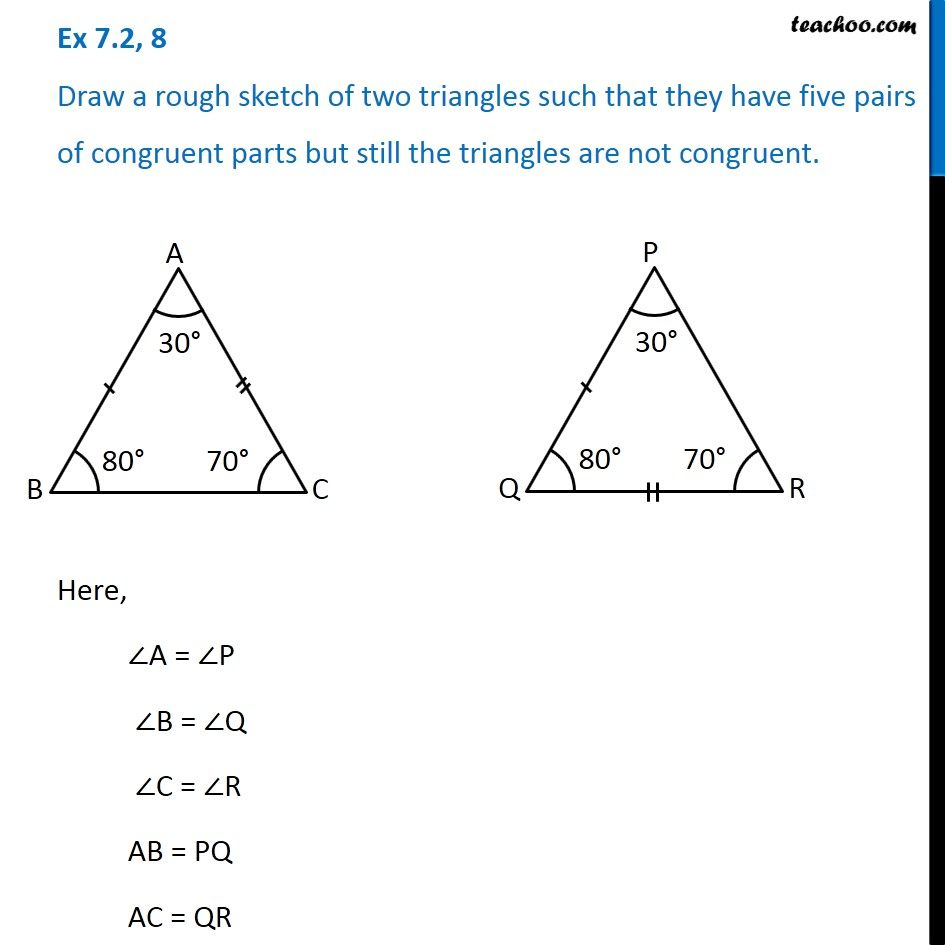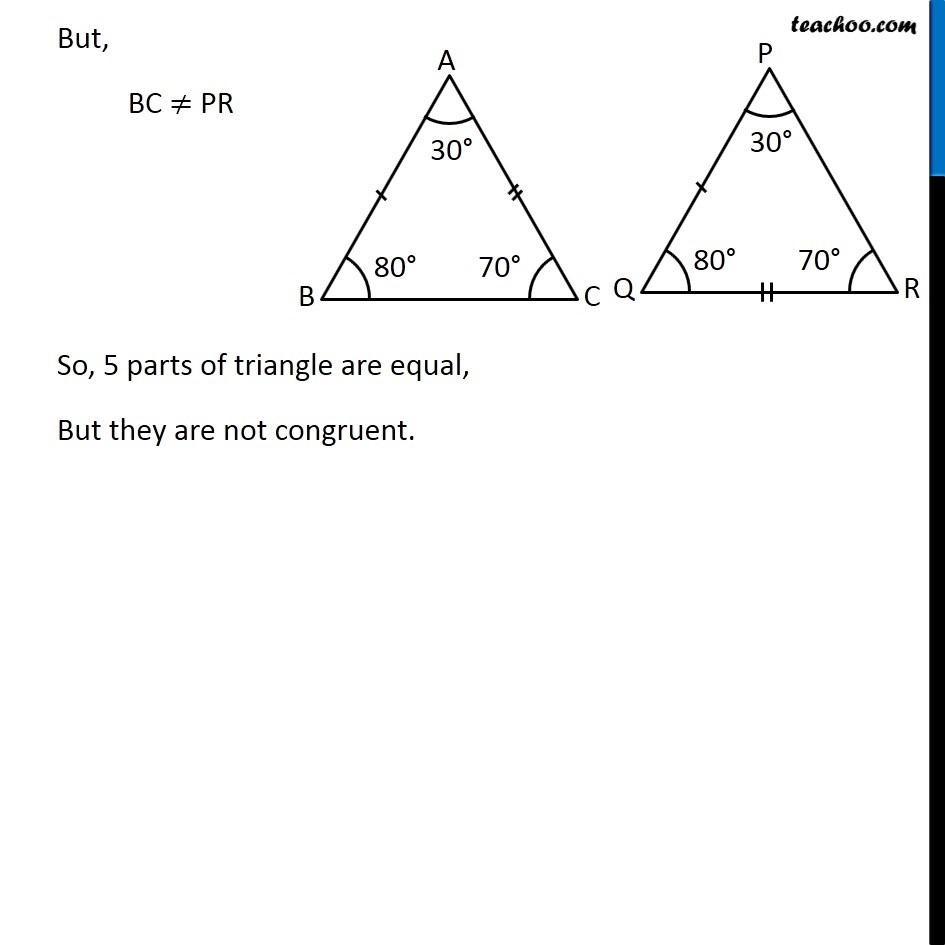1. Chapter 7 Class 7 Congruence of Triangles
2. Serial order wise
3. Ex 7.2

Transcript

Ex 7.2, 8 Draw a rough sketch of two triangles such that they have five pairs of congruent parts but still the triangles are not congruent.Here, ∠A = ∠P ∠B = ∠Q ∠C = ∠R AB = PQ AC = QR But, BC ≠ PR So, 5 parts of triangle are equal, But they are not congruent.

Ex 7.2